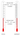# Temperature Units Converter# Temperature Units conversion

Fahrenheit to Celsius : subtract 32 and multiply by .5556 (or 5/9). Example: (50°F - 32) x .5556 = 10°C .
Celsius to Fahrenheit : multiply by 1.8 (or 9/5) and add 32. Example: (30°C x 1.8) + 32 = 86°F .
centigrade to Fahrenheit : simply double the degrees in C, and add 30 . for example 20deg C is app equal to 40 plus 30= 70 def F .
Fahrenheit to Centigrade : simply subtract (minus, or take away) 30 from degrees in F, and half it for example: 70 deg F i.e 70-30 = 40, divide 40 by 2 ( or half that amount) = 20 deg C .
1 Fahrenheit (°F) is equal to -17.22 Celsius (°C) ,
1 Fahrenheit (°F) is equal to 255.93 Kelvin (K)
1 Celsius (°C) is equal to 33.80 Fahrenheit (°F) ,
1 Celsius (°C) is equal to 274.15 Kelvin (K)